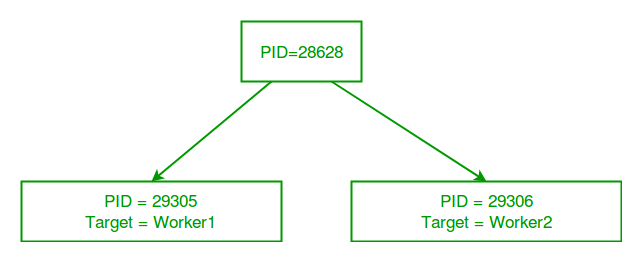Related Articles
Multiprocessing in Python | Set 1 (Introduction)
• Difficulty Level : Easy
• Last Updated : 09 Feb, 2018

What is multiprocessing?

Multiprocessing refers to the ability of a system to support more than one processor at the same time. Applications in a multiprocessing system are broken to smaller routines that run independently. The operating system allocates these threads to the processors improving performance of the system.

Why multiprocessing?

Consider a computer system with a single processor. If it is assigned several processes at the same time, it will have to interrupt each task and switch briefly to another, to keep all of the processes going.
This situation is just like a chef working in a kitchen alone. He has to do several tasks like baking, stirring, kneading dough, etc.

So the gist is that: The more tasks you must do at once, the more difficult it gets to keep track of them all, and keeping the timing right becomes more of a challenge.
This is where the concept of multiprocessing arises!
A multiprocessing system can have:

• multiprocessor, i.e. a computer with more than one central processor.
• multi-core processor, i.e. a single computing component with two or more independent actual processing units (called “cores”).

Here, the CPU can easily executes several tasks at once, with each task using its own processor.

It is just like the chef in last situation being assisted by his assistants. Now, they can divide the tasks among themselves and chef doesn’t need to switch between his tasks.

Multiprocessing in Python

In Python, the multiprocessing module includes a very simple and intuitive API for dividing work between multiple processes.
Let us consider a simple example using multiprocessing module:

 `# importing the multiprocessing module``import` `multiprocessing`` ` `def` `print_cube(num):``    ``"""``    ``function to print cube of given num``    ``"""``    ``print``(``"Cube: {}"``.``format``(num ``*` `num ``*` `num))`` ` `def` `print_square(num):``    ``"""``    ``function to print square of given num``    ``"""``    ``print``(``"Square: {}"``.``format``(num ``*` `num))`` ` `if` `__name__ ``=``=` `"__main__"``:``    ``# creating processes``    ``p1 ``=` `multiprocessing.Process(target``=``print_square, args``=``(``10``, ))``    ``p2 ``=` `multiprocessing.Process(target``=``print_cube, args``=``(``10``, ))`` ` `    ``# starting process 1``    ``p1.start()``    ``# starting process 2``    ``p2.start()`` ` `    ``# wait until process 1 is finished``    ``p1.join()``    ``# wait until process 2 is finished``    ``p2.join()`` ` `    ``# both processes finished``    ``print``(``"Done!"``)`
```Square: 100
Cube: 1000
Done!
```

Let us try to understand the above code:

• To import the multiprocessing module, we do:
```import multiprocessing
```
• To create a process, we create an object of Process class. It takes following arguments:
• target: the function to be executed by process
• args: the arguments to be passed to the target function

Note: Process constructor takes many other arguments also which will be discussed later. In above example, we created 2 processes with different target functions:

```p1 = multiprocessing.Process(target=print_square, args=(10, ))
p2 = multiprocessing.Process(target=print_cube, args=(10, ))
```
• To start a process, we use start method of Process class.
```p1.start()
p2.start()
```
• Once the processes start, the current program also keeps on executing. In order to stop execution of current program until a process is complete, we use join method.
```p1.join()
p2.join()
```

As a result, the current program will first wait for the completion of p1 and then p2. Once, they are completed, the next statements of current program are executed.

Let us consider another program to understand the concept of different processes running on same python script. In this example below, we print the ID of the processes running the target functions:

 `# importing the multiprocessing module``import` `multiprocessing``import` `os`` ` `def` `worker1():``    ``# printing process id``    ``print``(``"ID of process running worker1: {}"``.``format``(os.getpid()))`` ` `def` `worker2():``    ``# printing process id``    ``print``(``"ID of process running worker2: {}"``.``format``(os.getpid()))`` ` `if` `__name__ ``=``=` `"__main__"``:``    ``# printing main program process id``    ``print``(``"ID of main process: {}"``.``format``(os.getpid()))`` ` `    ``# creating processes``    ``p1 ``=` `multiprocessing.Process(target``=``worker1)``    ``p2 ``=` `multiprocessing.Process(target``=``worker2)`` ` `    ``# starting processes``    ``p1.start()``    ``p2.start()`` ` `    ``# process IDs``    ``print``(``"ID of process p1: {}"``.``format``(p1.pid))``    ``print``(``"ID of process p2: {}"``.``format``(p2.pid))`` ` `    ``# wait until processes are finished``    ``p1.join()``    ``p2.join()`` ` `    ``# both processes finished``    ``print``(``"Both processes finished execution!"``)`` ` `    ``# check if processes are alive``    ``print``(``"Process p1 is alive: {}"``.``format``(p1.is_alive()))``    ``print``(``"Process p2 is alive: {}"``.``format``(p2.is_alive()))`
```ID of main process: 28628
ID of process running worker1: 29305
ID of process running worker2: 29306
ID of process p1: 29305
ID of process p2: 29306
Both processes finished execution!
Process p1 is alive: False
Process p2 is alive: False
```
• The main python script has a different process ID and multiprocessing module spawns new processes with different process IDs as we create Process objects p1 and p2. In above program, we use os.getpid() function to get ID of process running the current target function.

Notice that it matches with the process IDs of p1 and p2 which we obtain using pid attribute of Process class.

• Each process runs independently and has its own memory space.
• As soon as the execution of target function is finished, the processes get terminated. In above program we used is_alive method of Process class to check if a process is still active or not.

Consider the diagram below to understand how new processes are different from main Python script:So, this was a brief introduction to multiprocessing in Python. Next few articles will cover following topics related to multiprocessing:

• Sharing data between processes using Array, value and queues.
• Lock and Pool concepts in multiprocessing

Next:

References:

This article is contributed by Nikhil Kumar. If you like GeeksforGeeks and would like to contribute, you can also write an article using contribute.geeksforgeeks.org or mail your article to contribute@geeksforgeeks.org. See your article appearing on the GeeksforGeeks main page and help other Geeks.